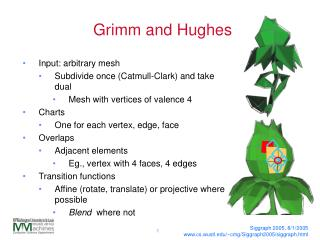DownloadDownload PresentationGrimm and Hughes

# Grimm and Hughes

Download Presentation## Grimm and Hughes

- - - - - - - - - - - - - - - - - - - - - - - - - - - E N D - - - - - - - - - - - - - - - - - - - - - - - - - - -
##### Presentation Transcript

1. Grimm and Hughes • Input: arbitrary mesh • Subdivide once (Catmull-Clark) and take dual • Mesh with vertices of valence 4 • Charts • One for each vertex, edge, face • Overlaps • Adjacent elements • Eg., vertex with 4 faces, 4 edges • Transition functions • Affine (rotate, translate) or projective where possible • Blend where not Siggraph 2005, 8/1/2005 www.cs.wustl.edu/~cmg/Siggraph2005/siggraph.html

2. Motivation • Maximize overlap • Three chart blend better than two • Co-cycle condition made > 3 hard • Affine transformations • (we got close) • Generalize spline construction process • Blend functions, not points Siggraph 2005, 8/1/2005 www.cs.wustl.edu/~cmg/Siggraph2005/siggraph.html

3. Charts • Vertex: Square • Always valence 4 • Edge: Diamond • Diamond shape determined by number of sides of adjacent faces • Face: N-sided unit polygon • Shrunk slightly Siggraph 2005, 8/1/2005 www.cs.wustl.edu/~cmg/Siggraph2005/siggraph.html

4. Overlaps • Vertex-face: corners • Vertex-edge: wedges • Edge-face: triangle • Edge-vertex: wedges • Face-vertex: corner quad • Face-edge: triangle Siggraph 2005, 8/1/2005 www.cs.wustl.edu/~cmg/Siggraph2005/siggraph.html

5. Transition functions • Edge-face: Affine • Translate, rotate, translate • Face-vertex: Projective • Square->quadrilateral • Edge-vertex: Composition Siggraph 2005, 8/1/2005 www.cs.wustl.edu/~cmg/Siggraph2005/siggraph.html

6. F1 Transition functions Vertex • Edge-vertex: F2 ? Siggraph 2005, 8/1/2005 www.cs.wustl.edu/~cmg/Siggraph2005/siggraph.html

7. Transition functions • C¥ continuous everywhere except blend area • Ck in blend area (determined by blend function) • At most three charts overlap anywhere • Reflexive: Use identity function • Symmetric: E-F, V-F both invertible • Co-cycle condition satisfied by blend function Siggraph 2005, 8/1/2005 www.cs.wustl.edu/~cmg/Siggraph2005/siggraph.html

8. f v e Abstract manifold Barycentric coords • “Glue” points that are related through transition functions • Each point appears in (at most) one each of a vertex, edge, and face chart f e v Siggraph 2005, 8/1/2005 www.cs.wustl.edu/~cmg/Siggraph2005/siggraph.html

9. Adding geometry • Blend and embed functions per chart • Fit to subdivision surface • 1-1 correspondence between manifold and dual mesh Siggraph 2005, 8/1/2005 www.cs.wustl.edu/~cmg/Siggraph2005/siggraph.html

10. Blend functions • Vertex: Spline surface basis function • Edge/face: Spline basis function “spun” in a circle • Inscribe • Partition of unity formed by dividing by sum of non-zero basis functions • Charts overlap sufficiently to guarantee sum non-zero Siggraph 2005, 8/1/2005 www.cs.wustl.edu/~cmg/Siggraph2005/siggraph.html

11. Embed functions • Spline patches • Fit to subdivision surface • One-to-one correspondence between dual mesh and charts • Implies correspondence between dual mesh and points in the chart Siggraph 2005, 8/1/2005 www.cs.wustl.edu/~cmg/Siggraph2005/siggraph.html

12. Plusses • Embed functions simple, well-behaved • Three-chart overlap • Transition functions (mostly) simple • Locality Siggraph 2005, 8/1/2005 www.cs.wustl.edu/~cmg/Siggraph2005/siggraph.html

13. Minuses • Blending composition function is ugly • Difficult to analyze • Large number of charts Siggraph 2005, 8/1/2005 www.cs.wustl.edu/~cmg/Siggraph2005/siggraph.html